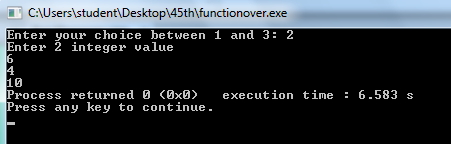## 20.7.13

C++ allows you to specify more than one definition for a function name or an operator in the same scope, which is called function overloading and operator overloading respectively.

You can have multiple definitions for the same function name in the same scope. The definition of the function must differ from each other by the types and/or the number of arguments in the argument list. You can not overload function declarations that differ only by return type.
Following is the example where same function print() is being used to print different data types:
```#include <iostream>
using namespace std;

class printData
{
public:
void print(int i) {
cout << "Printing int: " << i << endl;
}

void print(double  f) {
cout << "Printing float: " << f << endl;
}

void print(char* c) {
cout << "Printing character: " << c << endl;
}
};

int main(void)
{
printData pd;

// Call print to print integer
pd.print(5);
// Call print to print float
pd.print(500.263);
// Call print to print character
pd.print("Hello C++");

return 0;
}```
When the above code is compiled and executed, it produces following result:
```Printing int: 5
Printing float: 500.263
Printing character: Hello C++```
```

#include<iostream>
using namespace std;
class A
{
public:
int s,a,b,c;
void sum(int a, int b, int c)
{
cout <<a+b+c<<endl;
}
void sum(int a, int b)
{
cout<<a+b;
}
void sum(float a, float b)
{
cout<<a+b;
}
};
int main()
{
A aa;
int x,y,z,choice;
cout<<"Enter your choice between 1 and 3: ";
cin>>choice;
switch(choice)
{
case 1:

cout<<"Enter 3 interger value"<<endl;
cin>>x>>y>>z;
aa.sum(x,y,z);
break;
case 2:

cout<<"Enter 2 integer value"<<endl;
cin>>x>>y;
aa.sum(x,y);
break;

case 3:

float m,n;
cout<<"Enter 2 float value"<<endl;
cin>>m>>n;
aa.sum(m,n);
default:
break;
}
}

Output:Following is the example to show the concept of operator over loading using a member function. Here an object is passed as an argument whose properties will be accessed using this object, the object which will call this operator can be accessed using this operator as explained below:
#include <iostream>
using namespace std;

class Box
{
public:

double getVolume(void)
{
return length * breadth * height;
}
void setLength( double len )
{
length = len;
}

{
}

void setHeight( double hei )
{
height = hei;
}
Box operator+(const Box& b)
{
Box box;
box.length = this->length + b.length;
box.height = this->height + b.height;
return box;
}
private:
double length;      // Length of a box
double height;      // Height of a box
};
// Main function for the program
int main( )
{
Box Box1;                // Declare Box1 of type Box
Box Box2;                // Declare Box2 of type Box
Box Box3;                // Declare Box3 of type Box
double volume = 0.0;     // Store the volume of a box here

// box 1 specification
Box1.setLength(6.0);
Box1.setHeight(5.0);

// box 2 specification
Box2.setLength(12.0);
Box2.setHeight(10.0);

// volume of box 1
volume = Box1.getVolume();
cout << "Volume of Box1 : " << volume <<endl;

// volume of box 2
volume = Box2.getVolume();
cout << "Volume of Box2 : " << volume <<endl;

// Add two object as follows:
Box3 = Box1 + Box2;

// volume of box 3
volume = Box3.getVolume();
cout << "Volume of Box3 : " << volume <<endl;

return 0;
}

When the above code is compiled and executed, it produces following result:
Volume of Box1 : 210
Volume of Box2 : 1560
Volume of Box3 : 5400
```